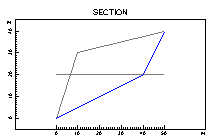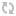# ACCECALC, CLASROCK, CLU_STAR, ROCK3D, ROTOMAP, ELETOM (c) geo *Dongle Emulator (Dongle Crack)*ACCECALC - Evaluation of the displacement of rock boulders subjected to dynamic forces, on the basis of a given accelerogram - determination, through pseudostatic analysis, of the acceleration that is necessary to bring the slope to limit equilibrium conditions - evaluation of the velocity and displacements in the direction of the sliding for each point of the considered accelerogram and calculation of the total displacement at the end of the seismic event in order to evaluate the stability of the slope under examination.
________________________________________
The program analyses the behaviour of the rock slopes under seismic conditions. It evaluates the displacements of a rock block subject to dynamic forces, on the basis of a given accelerogram.
The use of a calculation method based on a given accelerogram for rock slope stability analysis allows one to take into account the duration and the intensity of the accelerogram itself (this not being the case with the normally used limit equilibrium methods).
This allows a more realistic estimation of the effects of a seismic event on a potentially unstable rock block.
The program requires the shape of the rock slope face, the geomechanical parameters of the rock mass, the shape of the sliding surface and, as an option, of the water table.

In order to create a synthetic accelerogram, one should define the duration and maximum value of the foreseen seismic acceleration, the number of waves and their characteristic period. A recorded real accelerogram can also be used.
The synthesis of the accelerogram is carried out in four stages: selection of the wave characteristic period range; subdivision of this range into a number of intervals; generation of the sinusoidal waves with a phase obtained through random number generation; summation of the generated waves and determination of the acceleration values that make up the accelerogram.
The acceleration value that brings the slope to a limit equilibrium condition is calculated with a pseudostatic analysis; this acceleration is assumed to be the acceleration threshold beyond which velocity and relative displacements are generated.
When the duration of the event and the maximum acceleration is known, the program determines, through numerical integration of the accelerogram values, the velocities and displacements in the direction of the planar sliding surface.
The summation of the displacements calculated each time the accelerogram exceeds the critical acceleration determines the total displacement at the end of the seismic event. This constitutes a fundamental parameter with which the stability of the slope can be judged.
The same parameter set allows the program to generate different accelerograms, each of which can be used to calculate different final displacements of the potentially unstable blocks for further statistical analyses.

### Related news:

I.L.A., CE.CA.P., DIADIM, VERCAM, INSITU, LIQUITER, SID, WELL (c) GeoSoft *Dongle Emulator (Dongle Crack) for Eutron SmartKey*

Climatic Sumulation Program (for Subsurface Mines and Facilites) *Dongle Emulator (Dongle Crack) for Aladdin Hardlock*

Plaxis 3D 8.8 (c) PLAXIS BV *Dongle Emulator (Dongle Crack) for Aladdin Hardlock*